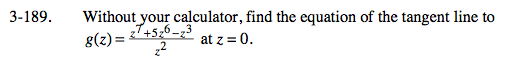### Home > CALC > Chapter Ch3 > Lesson 3.4.4 > Problem3-189

3-189.Write the tangent line in point-slope form: yy1 = m(xx1)

Find the point by evaluating g(0). Careful! Notice the denominator.

Though the denominator 'cancels out', there remains a hole in the graph at z = 0. Since there is no point, there is no line, and consequently no tangent line.

Step 2 would be to use g '(z) to find the slope at z = 0. However, since the tangent line does not exist, there is no reason to find its slope!